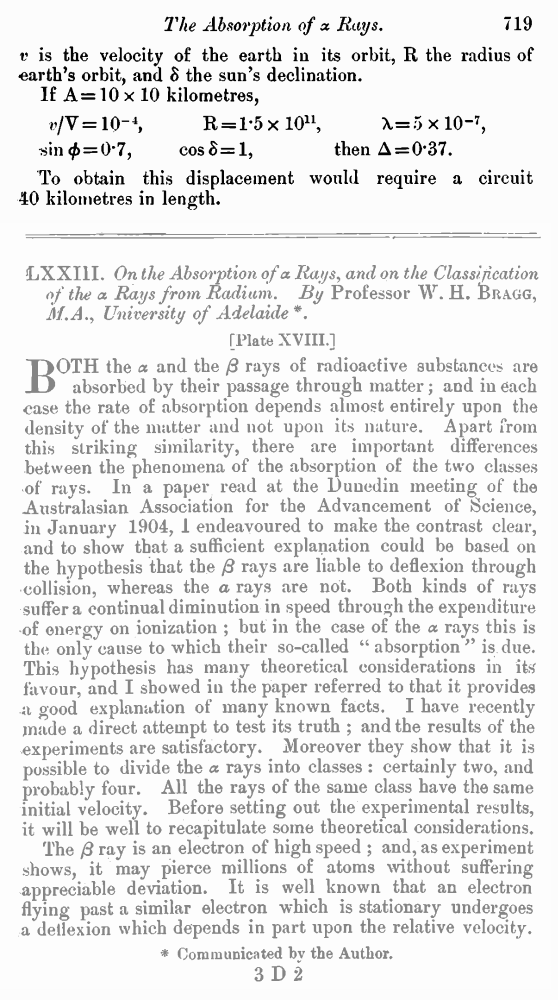# A. A. Michelson. Relative Motion of Earth and AEther // Phil. Mag. S. 6. Vol. 8. No. 48. Dec. 1904.

В начало   Другие форматы   <<<     Страница 719   >>>716  717  718  719 v is the velocity of the earth in its orbit, R the radius of earth’s orbit, and δ the sun’s declination. If A = 10 × 10 kilometres, v/V = 10–4, R = 1.5 × l011, λ=5 × 10–7, sin φ = 0.7, cos δ = 1, then Δ = 0.37. To obtain this displacement would require a circuit 40 kilometres in length.